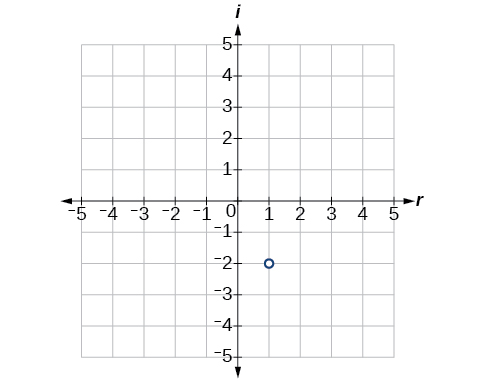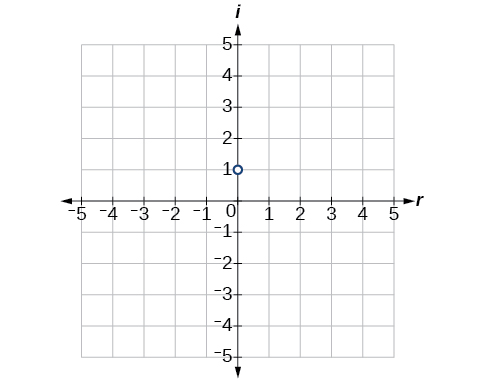# 2.4 Complex numbers  (Page 4/8)

 Page 4 / 8

## Simplifying powers of i

The powers of $\text{\hspace{0.17em}}i\text{\hspace{0.17em}}$ are cyclic. Let’s look at what happens when we raise $\text{\hspace{0.17em}}i\text{\hspace{0.17em}}$ to increasing powers.

$\begin{array}{l}{i}^{1}=i\hfill \\ {i}^{2}=-1\hfill \\ {i}^{3}={i}^{2}\cdot i=-1\cdot i=-i\hfill \\ {i}^{4}={i}^{3}\cdot i=-i\cdot i=-{i}^{2}=-\left(-1\right)=1\hfill \\ {i}^{5}={i}^{4}\cdot i=1\cdot i=i\hfill \end{array}$

We can see that when we get to the fifth power of $\text{\hspace{0.17em}}i,$ it is equal to the first power. As we continue to multiply $\text{\hspace{0.17em}}i\text{\hspace{0.17em}}$ by increasing powers, we will see a cycle of four. Let’s examine the next four powers of $\text{\hspace{0.17em}}i.$

$\begin{array}{l}{i}^{6}={i}^{5}\cdot i=i\cdot i={i}^{2}=-1\hfill \\ {i}^{7}={i}^{6}\cdot i={i}^{2}\cdot i={i}^{3}=-i\hfill \\ {i}^{8}={i}^{7}\cdot i={i}^{3}\cdot i={i}^{4}=1\hfill \\ {i}^{9}={i}^{8}\cdot i={i}^{4}\cdot i={i}^{5}=i\hfill \end{array}$

The cycle is repeated continuously: $\text{\hspace{0.17em}}i,-1,-i,1,$ every four powers.

## Simplifying powers of $\text{\hspace{0.17em}}i$

Evaluate: $\text{\hspace{0.17em}}{i}^{35}.$

Since $\text{\hspace{0.17em}}{i}^{4}=1,$ we can simplify the problem by factoring out as many factors of $\text{\hspace{0.17em}}{i}^{4}\text{\hspace{0.17em}}$ as possible. To do so, first determine how many times 4 goes into 35: $\text{\hspace{0.17em}}35=4\cdot 8+3.$

${i}^{35}={i}^{4\cdot 8+3}={i}^{4\cdot 8}\cdot {i}^{3}={\left({i}^{4}\right)}^{8}\cdot {i}^{3}={1}^{8}\cdot {i}^{3}={i}^{3}=-i$

Evaluate: $\text{\hspace{0.17em}}{i}^{18}$

$-1$

Can we write $\text{\hspace{0.17em}}{i}^{35}\text{\hspace{0.17em}}$ in other helpful ways?

As we saw in [link] , we reduced $\text{\hspace{0.17em}}{i}^{35}\text{\hspace{0.17em}}$ to $\text{\hspace{0.17em}}{i}^{3}\text{\hspace{0.17em}}$ by dividing the exponent by 4 and using the remainder to find the simplified form. But perhaps another factorization of $\text{\hspace{0.17em}}{i}^{35}\text{\hspace{0.17em}}$ may be more useful. [link] shows some other possible factorizations.

 Factorization of $\text{\hspace{0.17em}}{i}^{35}$ ${i}^{34}\cdot i$ ${i}^{33}\cdot {i}^{2}$ ${i}^{31}\cdot {i}^{4}$ ${i}^{19}\cdot {i}^{16}$ Reduced form ${\left({i}^{2}\right)}^{17}\cdot i$ ${i}^{33}\cdot \left(-1\right)$ ${i}^{31}\cdot 1$ ${i}^{19}\cdot {\left({i}^{4}\right)}^{4}$ Simplified form ${\left(-1\right)}^{17}\cdot i$ $-{i}^{33}$ ${i}^{31}$ ${i}^{19}$

Each of these will eventually result in the answer we obtained above but may require several more steps than our earlier method.

Access these online resources for additional instruction and practice with complex numbers.

## Key concepts

• The square root of any negative number can be written as a multiple of $\text{\hspace{0.17em}}i.\text{\hspace{0.17em}}$ See [link] .
• To plot a complex number, we use two number lines, crossed to form the complex plane. The horizontal axis is the real axis, and the vertical axis is the imaginary axis. See [link] .
• Complex numbers can be added and subtracted by combining the real parts and combining the imaginary parts. See [link] .
• Complex numbers can be multiplied and divided.
• To multiply complex numbers, distribute just as with polynomials. See [link] and [link] .
• To divide complex numbers, multiply both numerator and denominator by the complex conjugate of the denominator to eliminate the complex number from the denominator. See [link] and [link] .
• The powers of $\text{\hspace{0.17em}}i\text{\hspace{0.17em}}$ are cyclic, repeating every fourth one. See [link] .

## Verbal

Explain how to add complex numbers.

Add the real parts together and the imaginary parts together.

What is the basic principle in multiplication of complex numbers?

Give an example to show that the product of two imaginary numbers is not always imaginary.

Possible answer: $\text{\hspace{0.17em}}i\text{\hspace{0.17em}}$ times $\text{\hspace{0.17em}}i\text{\hspace{0.17em}}$ equals 1, which is not imaginary.

What is a characteristic of the plot of a real number in the complex plane?

## Algebraic

For the following exercises, evaluate the algebraic expressions.

If $\text{\hspace{0.17em}}y={x}^{2}+x-4,$ evaluate $\text{\hspace{0.17em}}y\text{\hspace{0.17em}}$ given $\text{\hspace{0.17em}}x=2i.$

$-8+2i$

If $\text{\hspace{0.17em}}y={x}^{3}-2,$ evaluate $\text{\hspace{0.17em}}y\text{\hspace{0.17em}}$ given $\text{\hspace{0.17em}}x=i.$

If $\text{\hspace{0.17em}}y={x}^{2}+3x+5,$ evaluate $\text{\hspace{0.17em}}y\text{\hspace{0.17em}}$ given $\text{\hspace{0.17em}}x=2+i.$

$14+7i$

If $\text{\hspace{0.17em}}y=2{x}^{2}+x-3,$ evaluate $\text{\hspace{0.17em}}y\text{\hspace{0.17em}}$ given $\text{\hspace{0.17em}}x=2-3i.$

If $\text{\hspace{0.17em}}y=\frac{x+1}{2-x},$ evaluate $\text{\hspace{0.17em}}y\text{\hspace{0.17em}}$ given $\text{\hspace{0.17em}}x=5i.$

$-\frac{23}{29}+\frac{15}{29}i$

If $\text{\hspace{0.17em}}y=\frac{1+2x}{x+3},$ evaluate $\text{\hspace{0.17em}}y\text{\hspace{0.17em}}$ given $\text{\hspace{0.17em}}x=4i.$

## Graphical

For the following exercises, plot the complex numbers on the complex plane.

$1-2i$$-2+3i$

$i$$-3-4i$

## Numeric

For the following exercises, perform the indicated operation and express the result as a simplified complex number.

$\left(3+2i\right)+\left(5-3i\right)$

$8-i$

$\left(-2-4i\right)+\left(1+6i\right)$

$\left(-5+3i\right)-\left(6-i\right)$

$-11+4i$

$\left(2-3i\right)-\left(3+2i\right)$

$\left(-4+4i\right)-\left(-6+9i\right)$

$2-5i$

$\left(2+3i\right)\left(4i\right)$

$\left(5-2i\right)\left(3i\right)$

$6+15i$

$\left(6-2i\right)\left(5\right)$

$\left(-2+4i\right)\left(8\right)$

$-16+32i$

$\left(2+3i\right)\left(4-i\right)$

$\left(-1+2i\right)\left(-2+3i\right)$

$-4-7i$

$\left(4-2i\right)\left(4+2i\right)$

$\left(3+4i\right)\left(3-4i\right)$

25

$\frac{3+4i}{2}$

$\frac{6-2i}{3}$

$2-\frac{2}{3}i$

$\frac{-5+3i}{2i}$

$\frac{6+4i}{i}$

$4-6i$

$\frac{2-3i}{4+3i}$

$\frac{3+4i}{2-i}$

$\frac{2}{5}+\frac{11}{5}i$

$\frac{2+3i}{2-3i}$

$\sqrt{-9}+3\sqrt{-16}$

$15i$

$-\sqrt{-4}-4\sqrt{-25}$

$\frac{2+\sqrt{-12}}{2}$

$1+i\sqrt{3}$

$\frac{4+\sqrt{-20}}{2}$

${i}^{8}$

$1$

${i}^{15}$

${i}^{22}$

$-1$

## Technology

For the following exercises, use a calculator to help answer the questions.

Evaluate $\text{\hspace{0.17em}}{\left(1+i\right)}^{k}\text{\hspace{0.17em}}$ for $\text{\hspace{0.17em}}k=4,8,\text{and}\text{\hspace{0.17em}}12.\text{\hspace{0.17em}}$ Predict the value if $\text{\hspace{0.17em}}k=16.$

Evaluate $\text{\hspace{0.17em}}{\left(1-i\right)}^{k}\text{\hspace{0.17em}}$ for $\text{\hspace{0.17em}}k=2,6,\text{and}\text{\hspace{0.17em}}10.\text{\hspace{0.17em}}$ Predict the value if $\text{\hspace{0.17em}}k=14.$

128i

Evaluate ${\left(\text{l}+i\right)}^{k}-{\left(\text{l}-i\right)}^{k}$ for $\text{\hspace{0.17em}}k=4,8,\text{and}\text{\hspace{0.17em}}12.\text{\hspace{0.17em}}$ Predict the value for $\text{\hspace{0.17em}}k=16.$

Show that a solution of $\text{\hspace{0.17em}}{x}^{6}+1=0\text{\hspace{0.17em}}$ is $\text{\hspace{0.17em}}\frac{\sqrt{3}}{2}+\frac{1}{2}i.$

${\left(\frac{\sqrt{3}}{2}+\frac{1}{2}i\right)}^{6}=-1$

Show that a solution of $\text{\hspace{0.17em}}{x}^{8}-1=0\text{\hspace{0.17em}}$ is $\text{\hspace{0.17em}}\frac{\sqrt{2}}{2}+\frac{\sqrt{2}}{2}i.$

## Extensions

For the following exercises, evaluate the expressions, writing the result as a simplified complex number.

$\frac{1}{i}+\frac{4}{{i}^{3}}$

$3i$

$\frac{1}{{i}^{11}}-\frac{1}{{i}^{21}}$

${i}^{7}\left(1+{i}^{2}\right)$

0

${i}^{-3}+5{i}^{7}$

$\frac{\left(2+i\right)\left(4-2i\right)}{\left(1+i\right)}$

$5-5i$

$\frac{\left(1+3i\right)\left(2-4i\right)}{\left(1+2i\right)}$

$\frac{{\left(3+i\right)}^{2}}{{\left(1+2i\right)}^{2}}$

$-2i$

$\frac{3+2i}{2+i}+\left(4+3i\right)$

$\frac{4+i}{i}+\frac{3-4i}{1-i}$

$\frac{9}{2}-\frac{9}{2}i$

$\frac{3+2i}{1+2i}-\frac{2-3i}{3+i}$

A laser rangefinder is locked on a comet approaching Earth. The distance g(x), in kilometers, of the comet after x days, for x in the interval 0 to 30 days, is given by g(x)=250,000csc(π30x). Graph g(x) on the interval [0, 35]. Evaluate g(5)  and interpret the information. What is the minimum distance between the comet and Earth? When does this occur? To which constant in the equation does this correspond? Find and discuss the meaning of any vertical asymptotes.
The sequence is {1,-1,1-1.....} has
how can we solve this problem
Sin(A+B) = sinBcosA+cosBsinA
Prove it
Eseka
Eseka
hi
Joel
June needs 45 gallons of punch. 2 different coolers. Bigger cooler is 5 times as large as smaller cooler. How many gallons in each cooler?
7.5 and 37.5
Nando
find the sum of 28th term of the AP 3+10+17+---------
I think you should say "28 terms" instead of "28th term"
Vedant
the 28th term is 175
Nando
192
Kenneth
if sequence sn is a such that sn>0 for all n and lim sn=0than prove that lim (s1 s2............ sn) ke hole power n =n
write down the polynomial function with root 1/3,2,-3 with solution
if A and B are subspaces of V prove that (A+B)/B=A/(A-B)
write down the value of each of the following in surd form a)cos(-65°) b)sin(-180°)c)tan(225°)d)tan(135°)
Prove that (sinA/1-cosA - 1-cosA/sinA) (cosA/1-sinA - 1-sinA/cosA) = 4
what is the answer to dividing negative index
In a triangle ABC prove that. (b+c)cosA+(c+a)cosB+(a+b)cisC=a+b+c.
give me the waec 2019 questionsByByByByByByBy